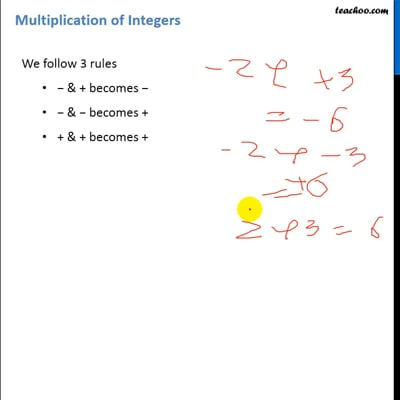Multiplication of Integers

Chapter 1 Class 7 Integers
Concept wise

• − & + becomes −
• − & − becomes +
• + & + becomes +

4 × −2

= −4 × 2

= −8

−8 × −3

= 8 × 3

= 24

9 × 4

= 36

10 × −2

= −20

−5 × −3

= 5 × 3

= 15This video is only available for Teachoo black users